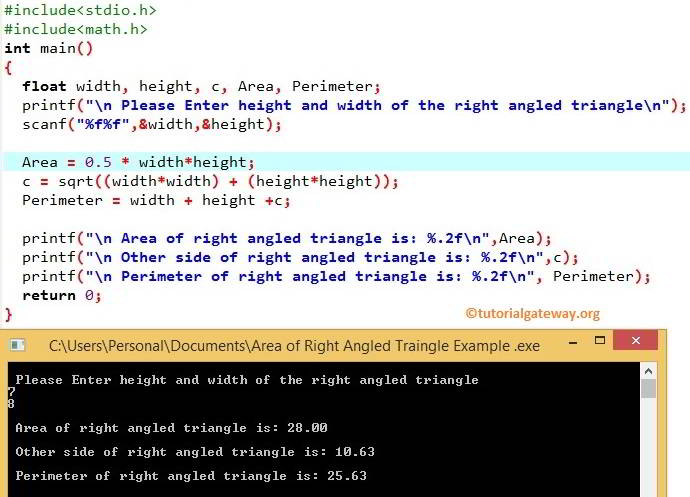# C Program to find Area of a Right Angled Triangle

How to write C Program to find Area of a Right Angled Triangle with example. Before we step into the Program to find Area of a Right Angled Triangle, Let see the definition and formula behind it.

## C Area of a Right Angled Triangle

If we know the width and height then, we can calculate the area of a right angled triangle using the below formula.

Area = (1/2) * width * height

Using Pythagoras formula, we can easily find the unknown sides in the right angled triangle.

c² = a² + b²

Perimeter is the distance around the edges. We can calculate the perimeter of a right angled triangle using the below formula

Perimeter = a + b+ c

## C Program to find Area of a Right Angled Triangle Example

This C Program allows the user to enter the width and height of the Right Angled Triangle. Using those values, we will calculate the Area and perimeter of the Right Angled Triangle.

```#include<stdio.h>
#include<math.h>
int main()
{
float width, height, c, Area, Perimeter;
printf("\n Please Enter height and width of the right angled triangle\n");
scanf("%f%f",&width, &height);

Area = 0.5 * width * height;
c = sqrt((width*width) + (height*height));
Perimeter = width + height + c;

printf("\n Area of right angled triangle is: %.2f\n",Area);
printf("\n Other side of right angled triangle is: %.2f\n",c);
printf("\n Perimeter of right angled triangle is: %.2f\n", Perimeter);
return 0;
}```Within the C Program to find Area of a Right Angled Triangle, the following statements will allow the User to enter the Width and Height of a right-angled triangle.

```  printf("\n Please Enter height and width of the right angled triangle\n");
scanf("%f%f",&width, &height);```

Next, we are calculating the area (The value of 1/2 = 0.5). So we used 0.5 * width*height as the formula

`Area = 0.5 * width * height`

In the next line of this Program, We are calculating the other side of a right-angled triangle using the Pythagoras formula C²=a²+b² , Which is similar to C = √a²+b²

`c = sqrt((width*width) + (height*height))`

Here we used sqrt() function in C Programming to calculate the square root of the a²+b². sqrt is the math function, which is useful to calculate the square root.

In the next line, We are calculating the perimeter using the formula.

`Perimeter = width + height + c`

Following printf statements will help us to print the Perimeter, Other side and Area of a right angled Triangle

```  printf("\n Area of right angled triangle is: %.2f\n",Area);
printf("\n Other side of right angled triangle is: %.2f\n",c);
printf("\n Perimeter of right angled triangle is: %.2f\n", Perimeter);```

NOTE: Please be careful while placing the open and close brackets, it may change the entire calculation if you place it wrong